# Effects of non-newtonian properties of blood flow on magnetic nanoparticle targeted drug delivery

Document Type : Research Paper

Authors

1 Department of Fluid Mechanics, Shiraz University, Shiraz, Iran

2 Department of Electronics and Communication Engineering, Shiraz University, Shiraz, Iran

3 Department of physiology, Shiraz University, Shiraz, Iran

Abstract

Objective(s): One applications of nanotechnology is in the area of medicine which is called nanomedicine. Primary instruments in nanomedicine can help us to detect diseases and used for drug delivery to inaccessible areas of human tissues. An important issue in simulating the motion of nanoparticles is modeling blood flow as a Newtonian or non-Newtonian fluid. Sometimes blood flow is simulated as a Newtonian fluid but actually blood has nature of non-Newtonian fluid.  Simulation of targeted drug delivery and motion of nanoparticles in the blood flow as Newtonian and non-Newtonian fluid flow is investigated in this paper
Materials and Methods: In this paper, the blood flow is modeled as both Newtonian and non-Newtonian fluid and the effects of each case on the motion of nanoparticles in blood flow and targeted drug delivery is investigated. The flow is modeled with finite volume method. The particle modeled with discrete phase model.
Results: Cross, Herschel-Bulkley and Power-law models are used for simulating the non-Newtonian blood flow. Numerical simulations show that trajectory of nanoparticle’s movement and the required time to pass the vessel by blood flow is variable for different models. According to obtained results, non-Newtonian Power-law and Herschel-Bulkley models have closely similar results but they have significant differences compared with Newtonian model.
Conclusion: According to the results, it is preferred in the simulation to model blood flow as a non-Newtonian fluid and uses one of Herschel- Bulkley or Power-law models. Otherwise the simulation is far different from real phenomena.

Keywords

#### Full Text

INTRODUCTION

The helpful application of nanotechnology is in the area of medicine which is called nanomedicine. Prevention and treatment of diseases are two roles of nanotechnology in the medicine science. This technology uses nanoinstruments and engineering nanostructures to see, recover, build and control of biology of human in the scale of molecules. Primary instruments in nanomedicine can help us to detect diseases, deliver drug, and distribute internal secretion in chronic illnesses and defects of body.

Advanced instruments such as nanorobots are used to perform surgical operation through human body. Nanorobots indeed, are robots which are in the size of nanometer. Some other use of nanomedicine can be suggested such as: generation of artificial tissues suitable for body, producing intelligent systems for detecting and processing diseases in body, therapy of difficult diseases such as the cancer, HIV, Hepatitis and health care with nanoequipments. As noted, one of the applications of nanotechnology is that nanoparticles can find receptors like cancer cells, trace the receptor, bind with them and finally release drug on the location of target cells .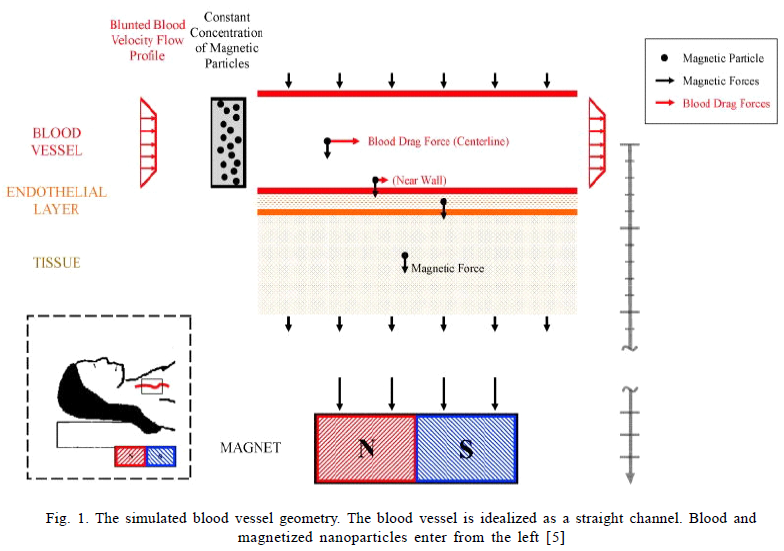Of course the process is very complex and several interactions among hydrodynamic forces, Brownian forces and adhesion forces affect the process. In addition to these effects, factors such as shape, size and material composing the nanoparticles have influence on the process. To develop nanomedicine, advanced numerical methods have been developed for modelling nanoparticle’s transport. These developments facilitate several activities to simulate nanoparticles’ motions. A great number of nanoparticle systems have a spherical shape and the magnitude of their diameters is an important parameter. For example, particles having diameters larger than 200 nanometers are filtered by spleen and are not allowed to cross. On the other hand, particles which have less than 10 nanometer diameters are filtered by kidney and leave the body. So that the best range of nanoparticle diameter is between 10 to 200 nanometers. Nanoparticles are delivered to human body in different ways such as: injection, inhalation or oral delivery. Among these methods which are commonly used, the method of delivering nanoparticle drug is the intravenous injection; after the injection, nanoparticles are transported along the blood flow [2-4].

Methods exist that first injects nanoparticles into vessel and then with a strong magnetic field reduce the velocity of nanoparticles and concentrate them to the specific location of body, Finally system of targeted drug delivery operates and drug is released at the vicinity of target cell. The simulated blood vessel geometry is shown in Fig 1.

Blood itself is a complex fluid which includes red blood cell (RBC) , white blood cell (WBC), plasma andetc. So nanoparticle transportation is affected by blood property . In some models and problems blood flow is being analyzed as a Newtonian fluid flow for simplicity [1,6], but in fact blood have the nature of a non-Newtonian fluid. In this article it has been shown that considering blood fluid flow as a Newtonian or non-Newtonian fluid flow affects the results. In this research, it has been tried to survey this effects in the simulation of nanoparticle transport. In the vicinity of the place where the targeted cells are located, nanoparticles are injected in blood flow and the flow carries them to the target cells. On this basis, type of interaction between blood flow and nanoparticles affects parameters such as: trajectory of nanoparticles transport and time of path duration in blood flow and we should consider this fact in simulations; it is important to have a true estimation and modeling to use the obtained data for real problem and human test, therefore system of targeted drug delivery will not operate well without this information and it may destroyed unharmed body cells. Several persons have worked on the effects that the blood fluid flow type has on the operation of targeted drug delivery [7-13]; but each of them has considered only one or two non-Newtonian models for the analysis of blood flow and to our knowledge a comprehensive comparison between different blood fluid models have not been yet performed .Some works have used CFD technique for blood flow simulation and this technique is very popular among researchers [14-20].  Using this technique or other computational techniques, one can simulate blood fluid as Newtonian and non-Newtonian fluid and survey blood flow behavior in human vessels such as arteries, veins and Capillaries [21-23].

For modeling the blood flow as non-Newtonian fluid, several models were presented. Some examples are:  Cross, Power-law, Herschel-Bulkey and etc. At the most of papers, one of these models was selected to investigate the problem. For the purpose of comparison, in this paper four models are considered for simulating blood fluid flow and the effects of each model on nanoparticle transport on blood flow and targeted drug delivery systems is investigated. In the subsequent parts, the mathematical and numerical modeling is represented.

In some works, nanoparticles’ concentration in blood fluid is studied under the influence of magnetic field, and so for simulation of blood flow, non-Newtonian model has been considered [24-26].

MATERIALS AND METHODS

Mathematical modeling

Two major parts can be considered for mathematical modeling; the first part can refer to the movement of particles and their related equations. The second part can refer to the equations of blood fluid flow which in this part, viscosity relations of each models are investigated. Particle movement and fluid flow have two viewpoints including Lagrangian and Eulerian. In fluid dynamics, the Lagrangian specification of the flow field is a method to surveying fluid motion where the observer follows an individual fluid particles as it moves through space and time. Plotting the position of an individual particle through time gives the path line of it. The Eulerian specification of the flow field is another method to surveying fluid motion that focuses on specific locations at space through which the fluid flows as time passes.  In general, both the Lagrangian and Eulerian viewpoints of the flow field can be applied in any observer’s frame of reference, and in any coordinate system used within the chosen reference frame. The DPM (discrete phase model) performs Lagrangian trajectory calculations for dispersed phases (particles, droplets, or bubbles), including coupling with the continuous phase. An alternative approach for particle tracking and discrete-phase calculations coupled with multiple reference frames is to track particles on the basis of absolute velocity instead of relative velocity.

Equations of motion for particles

Numerical simulation can predict the trajectory of a discrete phase particle by integrating the force balance on the particle, which is written in a Lagrangian reference frame. This force balance equates the particle inertia with the forces acting on the particle, and can be written (for the x direction in Cartesian coordinates particle force balance) as :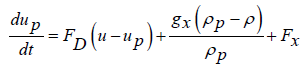(1)

Where Fx is an additional acceleration (force/unit particle mass) term, FD is drag force per unit particle mass which is calculated with drag coefficient relation: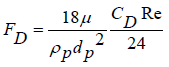(2)

Here, u is fluid phase velocity and up is particle velocity which it calculated with flow equations coupled with particle movement equations, ì is molecular viscosity of the fluid in order of 0.003 to 0.004 (kg/m.s) for Newtonian approach of blood fluid flow, ñ is fluid density which is 1056 (kg/m3) for blood fluid flow, is density of the particle, and dp is particle diameter. Re is relative Reynolds number, which is defined as: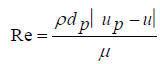(3)

Equation (1) incorporates additional forces (Fx) in particle force balance that can be important under special circumstances. The first of these circumstances is the ”virtual mass” force, which is the force required to accelerate the fluid surrounding the particle. This force can be written as: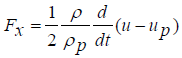(4)

The drag coefficient, CD, can be written as: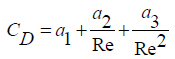(5)

Where a1, a2, and a3 are constants that are applied to smooth spherical particles over several ranges of  Re given by Morsi and Alexander , and can be written for other particle shapes as: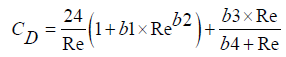(6)

Where there are the following values for the constants: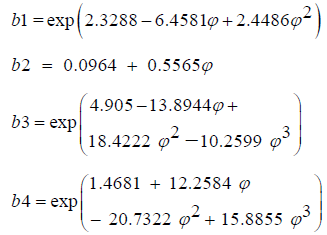Which is taken from Haider and Levenspiel . The shape factor, is defined as: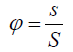(11)

Where s is the surface area of a sphere having the same volume as the particle, and S is the actual surface area of the particle. For sphere shape, F is equal to one. The Reynolds number is computed with the diameter of a sphere having the same volume. For sub-micron particles, a form of Stokes’ drag law is available . In this case, FD is defined as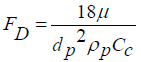(12)

The factor Cc is the Cunningham correction to Stokes’ drag law, which it can be calculated using the following  equation: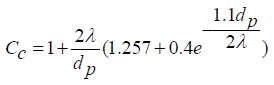(13)

Where l is the molecular mean free path where its value is around of 10-8.

Brownian Force

For sub-micron particles, the effects of Brownian motion can be optionally included in the additional force term. At microscale, the drag force acting on particles such as blood cells is significantly large compare to Brownian force, thus Brownian motion is negligible. While, at nanoscale Brownian force becomes a dominant force to drive nanoparticle under vascular flow or near to the vascular wall surface, where, the drag force acting on a nanoparticle is relatively small .

The components of the Brownian force are modeled as a Gaussian white noise process with spectral intensity Sn,ij given by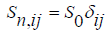(14)

Where dij is the Kronecker delta function, and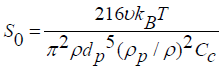(15)

T is absolute temperature of the fluid and for blood fluid flow in vascular is around 310 (k), í is kinematic viscosity, and kB is the Boltzmann constant which its value 1.38×10-23 (j/k). Amplitudes of the Brownian force components are of the following form: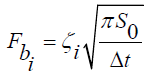(16)

Where zi are zero-mean, unit-variance-independent Gaussian random numbers. The amplitudes of the Brownian force components must be evaluated at each time step. The energy equation must be solved in order to the Brownian force to take effect.

Viscosity and shear stress equation

This part focuses on viscosity and shear stress equations to compare variation of viscosity versus rate of deformation, in four models of blood fluid flow.

Newtonian fluid flow model

For incompressible Newtonian fluids, the shear stress is proportional to the rate of deformation tensor (D), which can be written as: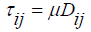(17)

Where D is defined by equation (18) and also calculated where the flow field is solved.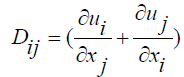(18)

m Is the viscosity, which is independent of rate of deformation tensor. In other words, for the Newtonian fluids viscosity is constant, which its value for blood is selected in the range of 0.003 to 0.004 (kg/m.s) .

Non-Newtonian fluid model

For non-Newtonian fluids, the shear stress can similarly be written in terms of a non-Newtonian viscosity h as follows: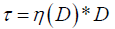(19)

In general, h(D) is a function of all three invariants of the rate-of-deformation tensor D, but usually used one or two of invariants to calculate the ç. In the non-Newtonian models available in this paper, h is considered to be a function of the shear rate  only.

Is related to the second invariant of D and is defined as: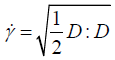(20)

Power law model

One of the most widely used forms of the general non-Newtonian constitutive relation is a power law model, which can be described as :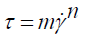(21)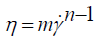(22)

Where his non-Newtonian apparent viscosity and mand nare power-law model constants. The constant, m, is a measure of the consistency of the fluid: the higher the m, the more viscous the fluid. nis a measure of the degree of non-Newtonian behavior: the greater the difference from the unity, the more pronounced the non-Newtonian properties of the fluid.

If n < 1, a shear thinning fluid is obtained, which is characterized by a progressively decreasing apparent viscosity with increasing shear rate. If n > 1, a shear-thickening fluid is obtained, in which the apparent viscosity increases progressively with increasing shear rate. When n = 1, a Newtonian fluid is obtained.

One of the obvious disadvantages of the power-law model is that it fails to describe the viscosity of many non-Newtonian fluids in very low and very high shear rate regions.

Cross model

The Cross model for viscosity is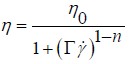(23)

Where h0 is zero-shear-rate viscosity,G is natural time (i.e., inverse of the shear rate at which the fluid changes from Newtonian to power-law behavior where its value is around 0.125) and n is power-law index. At an intermediate shear rate, the Cross model behaves like a power-law model.

Herschel-Bulkley Model

The Herschel-Bulkley model extends the simple power-law model to include a yield stress as follows :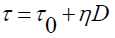(24)

Where t0 is the yield stress and assumed 17.5 mPa for this study. The Herschel-Bulkley model combines the effects of Bingham and power-law behavior in a fluid. For low strain rates ( ), the rigid material acts like a very viscous fluid with viscosity. As the strain rate increases and the yield stress threshold,t0 , is passed, the fluid behavior is described by a power law.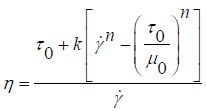(25)

Where k is the consistency factor which is assumed 8.972 and n is the power-law index.

The Herschel-Bulkley model also gives better fit for many biological fluids and food products than Newtonian model [34, 35].

Numerical simulation, results and discussion

CFD software based on finite volume was used in present numerical investigation to simulate nanoparticle motion in blood fluid flow. For the simulation of blood vessel, knowing that components of blood such as red blood cell (RBC) and white blood cell (WBC) tend to concentrate at the center of vessel and move on midsection of it , therefore cell free zone is created near the vessel wall. When nanoparticles are injected into blood vessel, they tend to move in the cell free zone, i.e. nanoparticles move near the blood vessel wall [1, 27]. Because of this, cell free zone is modeled in the simulation, and the motion of nanoparticles in this zone is studied. Another assumption is that nanoparticles move by blood flow velocity and also the interaction between particles, fluid and Brownian forces in the model has been considered. Continuum model can be used in cell free zone for diffusion of nanoparticles, and consequently calculations will be done faster than other models . So modeling of blood flow is very important and it is discussed in the mathematical modeling. In the first case, the blood flow is chosen as Newtonian fluid and nanoparticle motion is simulated. Range of viscosity for Newtonian model is about the order of 0.003 to 0.004 (kg/m.s) and  is selected equal to 0.003 (kg/m.s) [1, 36]. Blood flow velocity is assumed equal to v = 0.175 m/s. For this velocity, blood flow is laminar and does not sense the effects of turbulences .

The simulation of cell free region and flow domain  is 2d channel with dimension 5 µm× 15 µm which it have structured grid with 12230 elements. The quality of grid is investigated with the same case with 22450 elements. There were no differences between results of two cases. To validate the result, at first, Newtonian model has been considered. For this case, Newtonian simulation result has been validated with Samar Shah results . The trajectories of nanosphere particle in Newtonian blood fluid flow has been obtained from 20 independent simulations, and mean trajectory of these cases is shown in Fig. 2.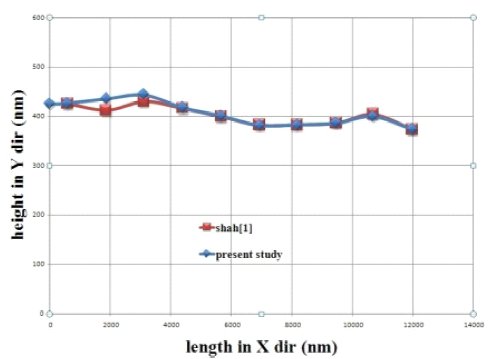Fig. 2. Mean trajectory of 20 trials for nanoparticle motion in 15×5 ìm cell free region in Newtonian blood fluid flow model
obtained in reference 1 and this study

It is observed that, maximum error between data is less than 5 percent. At present study, data is also compared with Shah’s data and results are compatible. Non-Newtonian models are simulated Based on the previous trend.  For the numerical simulation of blood flow as non-Newtonian fluid, primary data from references [21, 27, 36, 37] is used and it is as below:

Non-Newtonian index: n = 0.63, k = 16.1 mpa.s

The results shows that changing the property of fluid flow is affected on the parameters of the models. To show the sample, trajectory of a single nanoparticle and duration of passing this path are studied and simulated. The results can be seen in Fig. 3.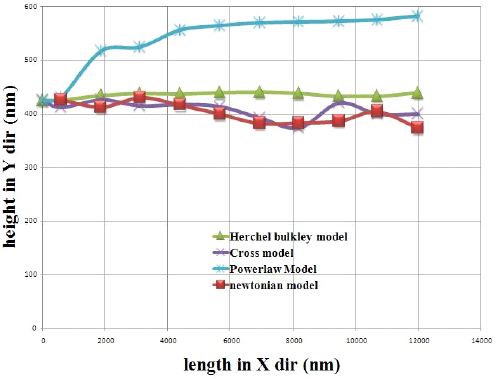Fig. 3. Comparing mean trajectory of nanoparticle motion in Newtonian and non-Newtonian blood flow in cell free region (15*5 ìm) of vessel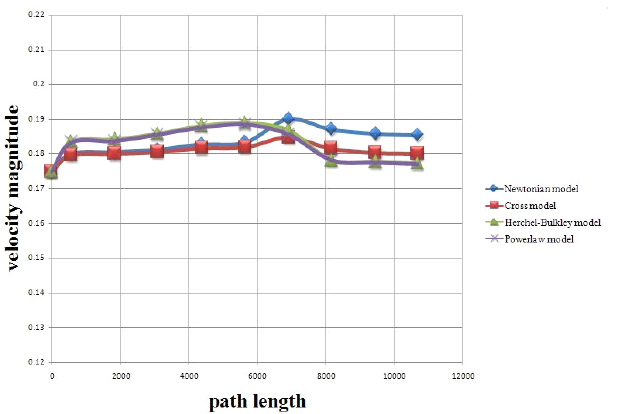Fig. 4. Velocity magnitude of particle along its path in blood flow models

It can be seen from the fig that with changing the fluid property, the trajectory of particle is changed too. So the path of particle is changed by the variation of blood flow properties. On the Table 1 the difference of duration of nanoparticle’s trajectory for Newtonian and non-Newtonian blood fluid flow is presented.

Based on the data tabulated in Table 1, it can be said that the difference between path durations corresponding to different models is in the order of millisecond.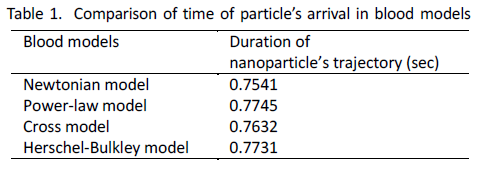On macroscopic scales, this variation is very small but on nanoscale this variation is remarkable and should be considered.

As it can be seen, when Newtonian blood fluid flow is used, time of path duration is less than other models.  If a non-Newtonian model like power law is used, it is more realistic. In this case time is longer than Newtonian model. Application of this time difference is where it concentrates the injected nanoparticles into the specific region of body.

If time approximation and location region of nanoparticles have an error relative to real state, so the strong magnetic field may damage the body tissues and can cause blood clots. Fig. 4 shows that, in the beginning of the particles injection into the blood flow, velocity of particle will be adopted by the velocity of fluid, But after a few milliseconds, because of interactions between particles and components of the blood, the velocity of particles a little bit increases. When interactions are decreased, the velocity of particles tends to be constant.

Also it should be mentioned that changes in the particle’s  velocity is very small but these changes in blood models is variable and can lead to a change in viscosity of model.

The approximation of the true tests is very important, and it also depends on choosing the correct model for blood flow. So it is suggested to use non-Newtonian models like Herschel-Bulkley for simulations instead of Newtonian model.

It can be said that in comparison, cross model and Newtonian model have similar behaviors and Power-law and Herschel-Bulkley models also behave similar for blood fluid flow in human body.

CONCLUSION

The present study shows a mathematical modeling for nanoparticle motion in blood fluid flow.

This work presents a numerical investigation on the nanoparticle motion in blood fluid flow based on nanoparticle targeted drug delivery systems which is adopted with real state. The effects of Newtonian and non-Newtonian fluid modeling are studied in detail with respect to flow patterns and particle movement.

The study shows that the rheological properties of blood can significantly affect the flow and particle phenomena. The results of particle motion pattern into Newtonian blood fluid flow for targeted drug delivery systems at present work are in good agreement with previous studies which have used Newtonian model. Comparison between different models and experimental data relative to blood flow shows that non-Newtonian approximation models are more accurate than Newtonian model, which is expected.

Numerical results shows that cross model behavior for blood flow is similar to Newtonian model but Power-law and Herschel- Bulkley models have different behaviors in targeted drug delivery systems. However, these differences are not so great but they are important on microscopic scale comparatively. Also it is suggested to use Power-law or Herschel- Bulkley model for simulation of blood fluid flow in targeted drug delivery systems and uses these two models instead of Newtonian model.

ACKNOWLEDGMENTS

We acknowledge Kavoshgar Khorshid research center faculties for their scientific and theoretical helps.

CONFLICT OF INTEREST

The authors confirm that this article content has not any conflicts of interest.

#### References

1.Shah S. Numerical simulation of particle adhesion dynamics for applications in nanomedicine and biosensing. University of Texas at Arlington; 2009.
2.Calvo P, Gouritin B, Chacun H, Desmaële D, D’Angelo J, Noel JP, Georgin D, Fattal E, Andreux JP, Couvreur P. Long-circulating pegylated polycyanoacrylate nanoparticles as new drug carrier for brain delivery. Pharm Res. 2001; 18(8): 1157-1166.
3.Kukowska-Latallo JF, Candido KA, Cao Z, Nigavekar SS, Majoros IJ, Thomas TP, Balogh LP, Khan MK, Baker JR Jr. Nanoparticle targeting of anticancer drug improves therapeutic response in animal model of human epithelial cancer. Cancer Res. 2005; 65(12): 5317-5324.
4.Heidel JD. Administration in non-human primates of escalating intravenous doses of targeted nanoparticles containing ribonucleotidereductase sub unit M2 siRNA. Proceedings of the National Academy of Sci of the USA. 2007; 104(14): 5715-5721.
5.Nacev A, Beni C, Bruno O, Shapiro B. The behaviors of ferromagnetic nano-particles in and around blood vessels under applied magnetic fields. J Magn Magn Mater. 2011; 323: 651–668.
6.Farokhzad O, Khademhosseini A, Jon S, Hermmann A, Cheng J, Chin Kiselyuk A, Teply B, Eng G, Langer R. Microfluidic System for Studying the Interaction of Nanoparticles and Microparticles with Cells. Anal Chem. 2014; 77: 5453-5459.
7.Shaw S, Murthy P, Pradhan SC. Effect of non-Newtonian characteristics of blood on magnetic targeting in the impermeable micro-vessel. J Magn Magn Mater. 2015; 322: 1037–1043.
8.Furlani EJ, Furlani EP. A model for predicting magnetic targeting of multifunctional particles in the microvasculature. J Magn Magn Mater. 2007; 312: 187–193.
9.Shaw S, Murthy P. Magnetic targeting in the impermeable microvessel with two-phase fluid model Non-Newtonian characteristics of blood. Microvasc Res. 2010; 80(2): 209-212.
10.Liu Y, Tan J, Shah S. A Hybrid Model for Nanoparticle Targeted Delivery in Blood Flow. ASME  First Global Cong on Nano Eng for Med and Bio. 2010; NEMB-13253.
11.Wiwatanapataphee B, Chayantrakom K, Wu YH. Mathematical Modeling and Numerical Simulation of Fluid-Magnetic Particle Flow in a Small Vessel. MMMAS. 2013; 401: 230-238.
12.Singh R, Lillard JW. Nanoparticle-based targeted drug delivery. Exp Mol Pathol. 2009; 86: 215–223.
13.Gentile F, Decuzzi P. Time dependent dispersion of nanoparticles in blood vessels. J Biomed Sci Eng. 2010; 03(05):517–524.
14.Manimaran R. CFD Simulation of Non-Newtonian Fluid Flow in Arterial Stenoses with Surface Irregularities. Int J Mechanical Aerospace Industrial Mechatronic and Manufacturing Eng. 2011; 73.
15.Dasilva E. CFD simulation an approach to evaluate cardio vascular dysfunction. Modeling Simulation & Optimization. 2009; 978-953-307-048-3.
16.Tokuda Y, Song MH, Ueda Y, Usuib A, Akita T, Yoneyama S, Maruyama S. Three-dimensional numerical simulation of blood flow in the aortic arch during cardiopulmonary bypass. Eur J Cardiothorac Surg. 2008; 33: 164 - 167.
17.Arslan N, Tuzcu V, Nas S, Durukan A. CFD modeling of blood flow inside human left coronary artery bifurcation with aneurysms. The 3rd European Medical and Biological Eng Conf. 2005; IFMBE Proc 11(1).
18.Jung J, Hassanein A. Three-phase CFD analytical modeling of blood flow. Med Eng & Phys. 2008; 30: 91–103.
19.Bui A, Manasseh R. Multi scale modeling of cerebral blood flow. 7th Int Conf on CFD in the Minerals and Process Industries. 2009; CSIRO Melbourne Australia.
20.Nikparto A, Firoozabadi B. Numerical study on effects of Newtonian and Non-newtonian blood flow on local hemodynamics in a multi-layer carotid artery Bifurcation. CMM. 2011.
21.Valencia A, Zarate A, Galvez M, Badilla L. Non-Newtonian blood flow dynamics in a right internal carotid artery with a saccular aneurysm. Int J Numer Methods Fluids. 2007; 50: 751-764.
22.Szpinda M. External diameters of the pulmonary arteries in human foetuses: an anatomical. Digital and statistical study. 2008; 67 (4): 240–244.
23.Taylor CA. Finite element modeling of blood flow: Relevance to atherosclerosis. Stanford University; 2000.
24.Habib MR, Ghasemi M. Numerical study of magnetic nanoparticles concentration in biofluid (blood) under influence of high gradient magnetic field. J Magn Magn Mater. 2011; 323: 32–38.
25.Blue LM, Sewell MK, Kim DH, Brazel CS. Fluid Dynamics and Heating of Magnetic Nanoparticles in Simulated Blood Vessels. American Physical Society. 2009; 61st Ann Meeting of the APS Division of Fluid Dynamics.
26.Nacev A, Beni C, Bruno O, Shapiro B. The behaviors of ferromagnetic nano-particles in and around blood vessels under applied magnetic fields. J Magn Magn Mater.  2011; 323: 651–668.
27.Kim S. A Study of Non-Newtonian Viscosity and Yield Stress of Blood in a Scanning Capillary-Tube Rheometer. Drexel University; 2002.
28.Morsi SA, Alexander AJ. An Investigation of Particle Trajectories in Two- Phase Flow Systems. J Fluid Mech. 1972; 55(2): 193-208.
29.Haider A, Levenspiel O. Drag Coefficient and Terminal Velocity of Spherical and Nonspherical Particles. Powder Technol. 1989; 58: 63-70.
30.Ounis H, Ahmadi G, McLaughlin JB. Brownian Diffusion of Sub micrometer Particles in the Viscous Sub layerJ Colloid Interface Sci. 1991; 143(1): 266-277.
31.Li A, Ahmadi G. Dispersion and Deposition of Spherical Particles from Point Sources in a Turbulent Channel Flow. Aerosol Sci and Tech. 1992; 16: 209-226.
32.Bird R, Amstrong RB, Hassager C. Dynamics of Polymeric Liquids. 1st ed. New York: Wiley; 1987.
33.Tang R, Hansong S, Dilhan M. Estimation of the parameters of Herschel–Bulkley fluid under wall slip using a combination of capillary and squeeze flow viscometers. Rheologica Acta. 2004; 43(1): 80–88.
34.Siddiqui SU, Verma NK, Gupta RS. A mathematical model for pulsatile flow of Herschel-Bulkley fluid through stenosed arteries. J Sci & Tech. 2010; 5: 4-22.
35.Maruthi K. Flow of Herschel-Bulkley fluid through an inclined tube of non-uniform cross-section with multiple stenoses. Arch Mech. 2008; 60(2): 161-172.
36.Suleyman S. Computational studies of steering nanoparticles with magnetic gradients. Northeastern University; 2011.
37.Lenz C, Rebel A, Waschke KF, Koehler RC, Frietsch T. Blood viscosity modulates tissue perfusion: sometimes and somewhere. Transfus Altern Transfus Med. 2008; 9(4): 265–272.